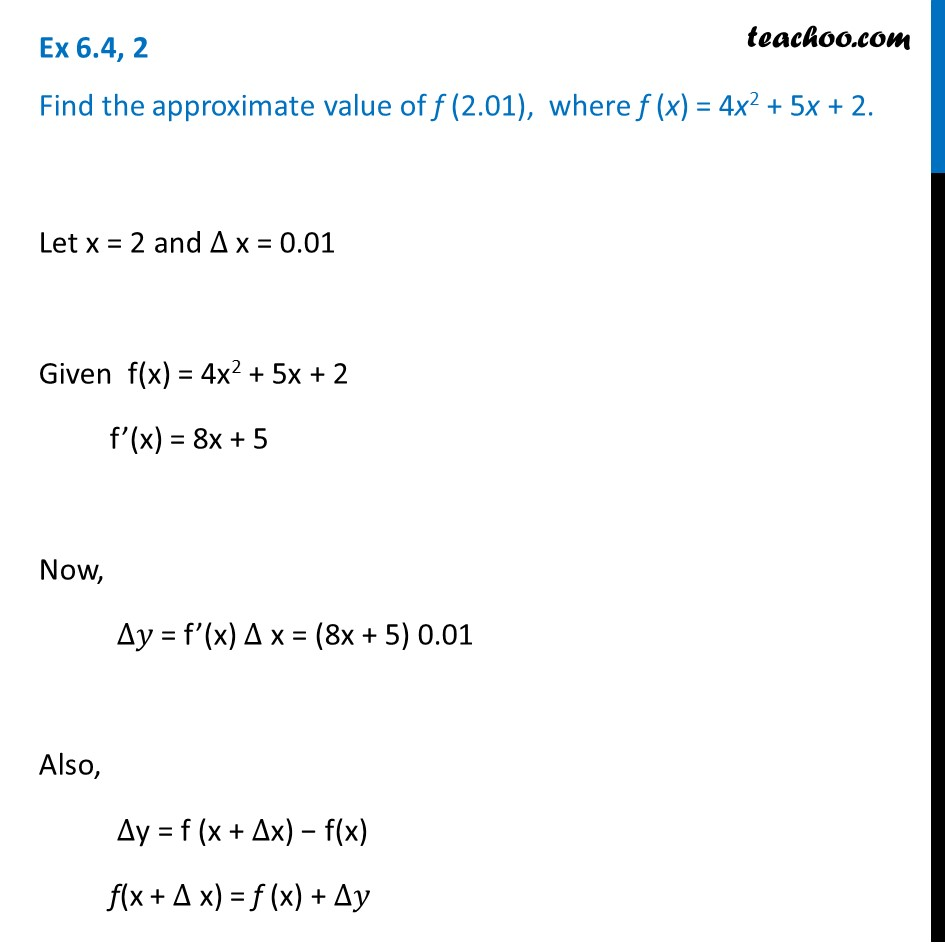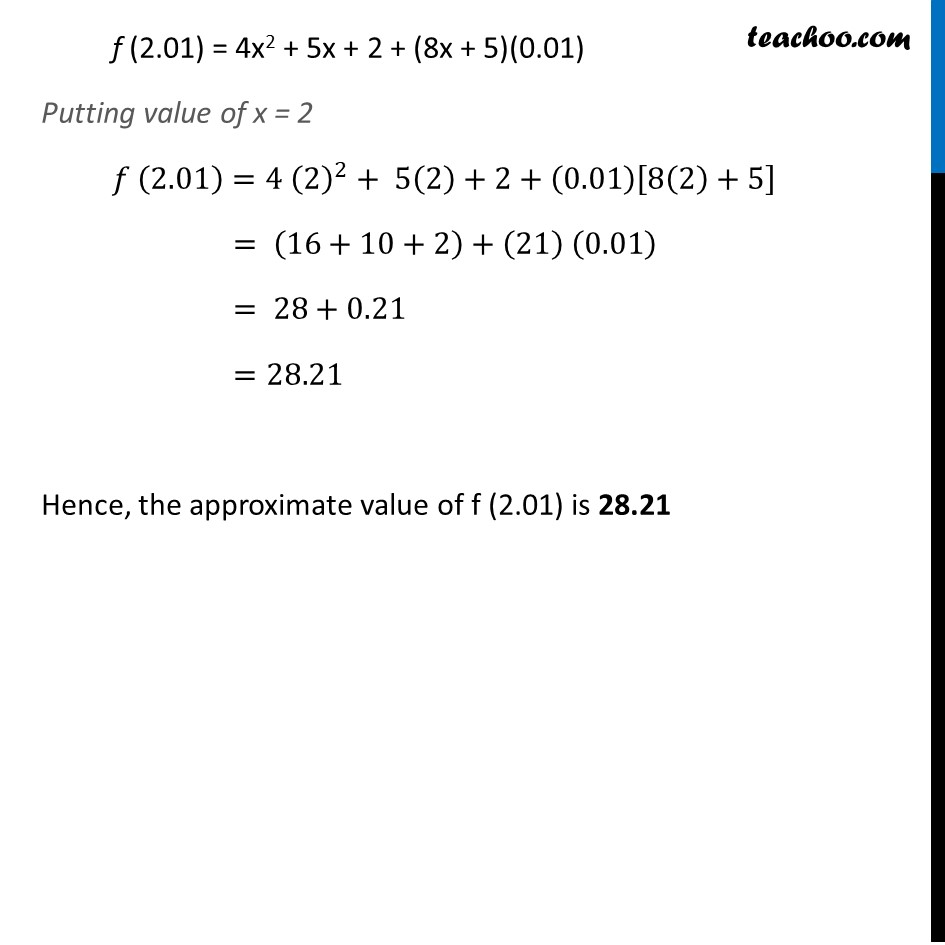Approximations (using Differentiation)

Chapter 6 Class 12 Application of Derivatives
Serial order wiseLearn in your speed, with individual attention - Teachoo Maths 1-on-1 Class

### Transcript

Question 2 Find the approximate value of f (2.01), where f (x) = 4x2 + 5x + 2.Let x = 2 and ∆ x = 0.01 Given f(x) = 4x2 + 5x + 2 f’(x) = 8x + 5 Now, ∆𝑦 = f’(x) ∆ x = (8x + 5) 0.01 Also, ∆y = f (x + ∆x) − f(x) f(x + ∆ x) = f (x) + ∆𝑦 f (2.01) = 4x2 + 5x + 2 + (8x + 5)(0.01) Putting value of x = 2 𝑓 (2.01)=4 (2)^2+ 5(2)+2+(0.01)[8(2)+5] = (16+10+2)+(21) (0.01) = 28+0.21 =28.21 Hence, the approximate value of f (2.01) is 28.21